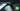# Hackerrank | Solution of Time Conversion in Golang

September 11th, 2018
|

In this one we will solve the last question from warmup section from hackerrank. Time Conversion. A quick and easy one, which only requires some formatting. Let's dive right into it.

# Problem Statement

The question can be found at this link. The problem statement states that we are given a time in 12-hour format i.e. AM-PM format and we have to convert it into military (24 hour) time format. Couple of things to note here is

• This problem can be solved in two ways, either using some string manipulation or some formatted input and printing. We will use the second way here, it's easier and smaller.
• There are two edge case i.e. when clock hits 12.

# Challenges

• Choose our preferred language as golang on hackerrank. The moment we do that, we get some 50-60 lines of code which are very unfamiliar to someone who is new to language.
• Handling the edge cases.

# Solution

This is the template you get on hackerrank for this problem statement.

``````    package main

import (
"bufio"
"fmt"
"io"
"os"
"strings"
)

/*
* Complete the timeConversion function below.
*/
func timeConversion(s string) string {
/*
*/

}

func main() {

outputFile, err := os.Create(os.Getenv("OUTPUT_PATH"))
checkError(err)

defer outputFile.Close()

writer := bufio.NewWriterSize(outputFile, 1024 * 1024)

result := timeConversion(s)

fmt.Fprintf(writer, "%s\n", result)

writer.Flush()
}

if err == io.EOF {
return ""
}

return strings.TrimRight(string(str), "\r\n")
}

func checkError(err error) {
if err != nil {
panic(err)
}
}``````
• First go ahead and delete everything in the pre-existing template.
• Let's write down our main wrapper function.
``````package main
import "fmt"

func main(){

}``````

Before jumping into any of the code logic, let's first discuss how are going to take input, and how many variables we need. So looking closely at the input `xx:xx:xxAM or xx:xx:xxPM`, xx is an integer , we have three of those, which are separated by `:` and the last one has a suffix attached to it. So we need three integers, and one string variable to hold our input, and take our input in a formatted fashion. Let's go ahead and handle that.

``````    ...
var h,m,s int
var suffix string

fmt.Scanf("%d:%d:%d%s",&h,&m,&s,&suffix)``````

Here we declare three integers and one string variable. Next we take one line of input. `%d` specifies an integer, three integers separated by colons and then followed by a string, that would take our suffix i.e. AM or PM. Now let's discuss the logic. First let's agree that the minute and second part of the time will always be the same no matter what the time is, it's only the hour part that will change. So we have two cases here. First is AM and second is PM. In case of AM if the hour is 12, it will become `00` else it will be same, for example, 2 o'clock in the morning is same in both the clock formats. Now for the case of PM, if the hour is not equal to 12, we will add 12 to it. For an example, 2 o'clock in the after noon is 14 o'clock in military clock format. Those are all the logic we need to solve this problem, next we will just format and print the results. Let's look at the code now.

``````	...
if suffix == "AM" && h == 12 {
h = 0
}
if suffix == "PM" && h != 12 {
h += 12
}
fmt.Printf("%02d:%02d:%02d",h,m,s)``````

So here we have our logical blocks, and the formatted print. `%02d` represents that it will be an integer in length 2, if the length is less than 2, prefix it with a zero. So That is all we need. Now your complete code should look like

``````package main
import "fmt"

func main(){
var h,m,s int
var suffix string

fmt.Scanf("%d:%d:%d%s",&h,&m,&s,&suffix)

if suffix == "AM" && h == 12 {
h = 0
}
if suffix == "PM" && h != 12 {
h += 12
}
fmt.Printf("%02d:%02d:%02d",h,m,s)

}``````

let's review what are the highlights to take away from this question. We could have solved this question using string manipulation as well as using formatted input and output methods. We went for the latter because as you can see, it made the code much smaller and simpler. Once we got the inputs in the desired formats we wanted, it was just a matter of simple logic and formatted printing. This was the last question from hackerrank warmup section, if hackerrank adds anymore question to this section, I will add a solution on my blog and upload a video for it, but for now, we are done with this section and will move to implementation section. This is it for this one, complete source code for this post can be found on my Github Repo. Will see you in the next one.